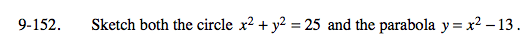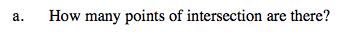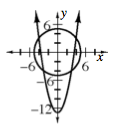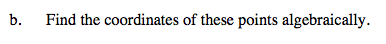### Home > A2C > Chapter 9 > Lesson 9.3.2 > Problem9-152

9-152.Look at the graph at right.

4 pointsSubstitute x2 − 13 into the first equation.

x2 + (x2 − 13)2 = 25

Solve for x.

x4 − 25x2 + 144 = 0

Factor the equation.

(x2 − 16)(x2 − 9)

(x − 4)(x + 4)(x − 3)(x + 3)

x = ±4, ±3

Now substitute in these x values to find the y.